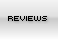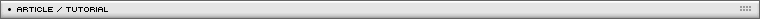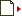Collision detection
[ September 18, 2003 ] by Mad Sci
Different collision-detection techniques: grid-based method; "hitTest()" method; "getBounds()" method; distance approximation; array-based method.

Collision detection is the heart and the soul of any computer game. Basically we have 2 types of collision detection:

1. collision with non-moving, background objects like for example walls or platforms;
2. collision with moving objects, for example bullets, enemies, etc.

In general mastering the aspects of collision detection will greatly improve the way you build your games and especially the speed of the games themselves.
So what is EXPLOSIVE GROWTH?. Lets take this example. On the stage we have 2 movie clips. Clip A and Clip B and we want to test if they are touching eachother. Basicaly this means that we have to perfom 2 collision detections. First Clip A checks if it touches Clip B, and then the opposite. Obviously this is redundand: if Clip A is thouching Clip B that means that B is touching A as well. So having n number of objects you have to perfom n2 collision tests, but obviously you will not do collision of the object with itself, so you eliminate n tests, and because "Object A touching Object B" = "Object B touching Object A", you discard half of the collision tests. Now you get a formula: Number_Collisions = (n2-n)/2. Now try to calculate the Number_Collision which should be performed for 3 objects and for 10 objects: n=3 and n=10. So how do we get around this? Number_Collision detection could be lowered by the rules of the game. As a game developer you are the one to decide which objects will collide and which objects will not collide.

Let's see some collision detection techniques.

GRID BASED METHOD

This method of collision detection is fast because it does not use movie clips, and it's very accurate. It is based on a string representation of your stage. Follow this example.
Create a movie of 300x300 pixels. Go to Edit Grid and make it 10x10 pixels and then show the grid. Now on the stage you see 30 rows and 30 collums. Each square represents a tile, in our case 10x10 pixels tiles. When we move our sprite (hero) we will be using step of 10. Thus the hero will jump from tile to tile; the hero sprite itself has a size of 10x10 pixels and a position on the stage in a way that it fits in one grid square exactly.

Define a string for each row, in there's a char for each column:

row01="XXXXXXXXXXXXXXXXXXXXXXXXXXXXXX"
row02="XOOOOOOOOOOOOOOOOOOOOOOOOOOOOX"
row03="XOOOOOOOOOOOOOOOOOOOOOOOOOOOOX"
row04="XOOOOOOOOOOOOOOOOOOOOOOOOOOOOX"
row05="XOOOOOOOOOOOOOOOOOOOOOOOOOOOOX"
...
row29="XOOOOOOOOOOOOOOOOOOOOOOOOOOOOX"
row30="XXXXXXXXXXXXXXXXXXXXXXXXXXXXXX"

where X represents non-walkable tiles, O walkable tiles.

When moving the hero UP and DOWN we will change the Row number, like row1, row2 etc. When we move the hero LEFT or RIGHT we will extract a substring from the default row. For example:

1. in the onClipEvent (load) statement specify the start up coordinates of the hero: row = 5 and col = 10, where "row" is row number, "col" is the column number;
2. create a function which will look in the table for collision:
```function wall (r,c)
{
check = substring (eval("row" + r), c, 1); // extract the letter from the string
if (check == "X") return false;
else return true; //check if it is X
}```

To call the function:

```if (Key.isDown (Key.UP))
{
walk = wall(Math.floor(row-1), Math.floor(col));
//note the math.floor thingie: we work with integers...

if (walk)
{
this._y -= 10;
row -= 1;
}
}```

Now assume that we are currently at row 5, column 10 or as we set it up in onClipEvent(load){ row=5; col=10; }.
The user presses the UP key.

1. we submit the following values to the function walk: row = 4, col = 10 -> walk(row-1, col);
2. the function looks in the table: substring("row" + r, c, 1), which means: row 4, 10th letter = O.

row04="XOOOOOOOOOOOOOOOOOOOOOOOOOOOOX"
row05="XOOOOOOOOOOOOOOOOOOOOOOOOOOOOX"

In this case the function wall will return false since check is not X, so we move up with 10px, and we move the current row up with one...

What are the draw backs of this approach? Basicaly building the grid table, making corrections on the fly as you program and design the game. To overcome this you must build a Game Engine which will allow you to position, move tiles around and generate the look up table for you.

hitTest() BASED METHOD

hitTest() is a fast and easy way to detect collisions with the walls. The draw back is that it uses movie clip. We use the following format to check for collision: on the stage you put all non-walkable tiles in a layer called non-walkable. Under that layer you put the walkable tiles. Now select all tiles in the non-walkable layer and convert it to movie clip and give it instance name "back".

In the hero sprite put this code wraped in onClipEvent(enterFrame):

```if (Key.isDown (Key.UP))
{
if (!_root.back.hitTest(this._x, this._y-10, true)) this._y -= 10;
}```

This is the point based hitTest() function. Note that again we look ahead or where the sprite will be (this._y-10) and if the hitTest returns false we will move there with step of 10 again.

COLLISIONS WITH BONUS ITEMS

In game like PacMan for example you have to walk around and eat pills. In our case each pill will be a movie clip (drawback) and each pill will look for collision with the hero sprite. So in the pill movie you can put:

if (hitTest(_root.hero)) removeMovieClip(this);

In this case we use the bounding box hitTest() method. As you eat the pills and remove them from the stage the game will increase its speed because less movies will be on the stage. The same rule could be applyed for any pick up item or bonus item in general. You can also increase the score before removing the clip usign:

_root.score += 50 for example, and then removeMovieClip(this);

COLLISIONS USING DISTANCE APPROXIMATION (DA)

Basicaly DA is based on Pythagoras theorem: a2+b2=c2 or c=Math.sqrt(a2+b2), where c is the distance between the two objects, a is the distance on the x-axis and b the distance on the y-axis. In Flash this could be done using this approach: make 2 circle movie clips on the stage and name them M1 and M2.

In frame 1 on the main time line:

```R1 = _root.m1._width/2; //radius of M1
R2 = _root.m2._width/2; //radius of M2
radius = r1 + r2; //this sum should be substracted from the c
Dx = _root.m1._x - _root.m2._x; //the difference between the _x
Dy = _root.m1._y - _root.m2._y; //the difference between the _y
a = Dx * Dx;
b = Dy * Dy;
c = Math.floor(Math.sqrt(a+b) - radius); //if 0 or less the clips are touching}```

Of cource it would be much more simple to use hitTest() in this case because the clips are with reqular shapes: circles. Some times you want the coordinates of a movie clips to be alligned like for example when you want to climb a ladder the hero should be positioned right in the middle. The problem is that you cannot use simply: if (ladder_x = hero_x) {...}; the reason is that the hero is moving with a fixed step of, let's say, about 5 and a difference of one pixel will return false statement. To overcome this you can set up a small alignment array: dif = ladder_x - hero_x
Then you can use if(diff >- && diff < 5) {...}: in this case the ladder will look for collision only if the difference between the _x coordinates are in the range of +- 5 pixels. Now if we assume that you have many ladders at any moment they will return some variables to the hero even if the hero is not actually colliding with the ladder, so we use hitTest() first to indicate that the hero is colliding with a particular ladder and then we use difference approximation to check if he is actually alligned in the middle.

COLLISIONS USING getBounds() METHOD

getBounds() will basically return the LEFT, RIGHT, TOP, BOTTOM bounds of an object, which are the coordinates of the bounding box. It is relatively easy to use. So take your movie clip, get the actions panel and paste:

```OnClipEvent(enterFrame)
{
my_bounds = new Object(); //create a new object
my_bounds = this.getBounds(_root); //get the bounds
}```

Now to get the left bounds for example you can use: left = my_bounds.Xmin; and for the right: right=my_bounds.Xmax; etc.
For example if you want to see if there is a ground right under the hero you can use getBounds() together with hitTest(): if(_root.back.hitTest(this._x, my_bounds.Ymax + 2)){...}, where "back" is the name of the backgrownd movie clip holding the platforms.

In the hero MC:

```onClipEvent(enterFrame)
{
bounds = new Object();
bounds = this.getBounds(_root);
if(_root.back.hitTest(this._x, bounds.Ymax + 2)) _root.show = "collision";
else _root.show = "NOcollision";
}
// bounds.Ymax+2 means 2 pixels below the lower boundary of the hero```

COLLISIONS USING ARRAY BASED METHOD

This part is purely theoretical. I personally have not experimented with it.

The Scene could be split in different squares forming a grid, and the coordinates of each cell can be put into an array. The idea is that if 2 objects are within one an the same grid cell they must be colliding, like 2 objects in the real life cannot occupy one and the same space at particular moment of time. I personally assume that this could take up a lot of processing power and slow down the game. It coul be used to eliminate unnecessary collision detections, also this could be used for a collision detection between clips with irregular shapes.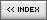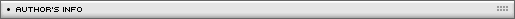Name: Mad Sci Location: N/A Age: N/A Flash experience: N/A Job: N/A Website: http://mad-sci.lazoom.com/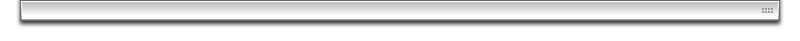| Homepage | News | Games | Articles | Multiplayer Central | Reviews | Spotlight | Forums | Info | Links | Contact us | Advertise | Credits || www.smartfoxserver.com | www.gotoandplay.biz | www.openspace-engine.com | gotoAndPlay() v 3.0.0 -- (c)2003-2008 gotoAndPlay() Team -- P.IVA 03121770048